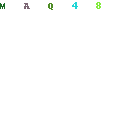## The Books on a Table Scenario - Newton's Third LawThe Problem:

Students have misconceptions about static forces and Newton’s 3rd Law (every action has an equal and opposite reaction).

Example:

Imagine there is a stack of books on a table. A picture of that scenario is presented on a science test. Students are asked to draw the (Newtonian) forces that are occurring in that situation. The majority of students typically draw the force of gravity, pointing down from the books, but they forget that according to Newton's third law, there is an equal and opposite force that the table exerts on the books in the upward direction. Students do not believe or recognize that the table is pushing back up at the books with a force equal and opposite to the force of gravity from the books

Instructional Design Strategies:

One must use one or more pedagogical strategies to help students understand the forces involved in the book-table system and recognize the 'hidden' force that the table exerts on the books. Below are some examples.

 Strategy Example Verbal, Symbolic Inscriptions, Direct Instruction Present a verbal Newtonian description of the forces occurring in the system. Explain Newton’s laws with phrases and symbols such as “action, reaction” and “F=ma.” Diagrammatic Visual Representations Construct a diagram of the books and table, and draw arrows to construct a free-body diagram of the static forces involved in the system. Perceptual Magnification Place a mirror on the table, shine a laser towards it at an angle such that it reflects onto the wall. When the books are placed on the table the laser point visibly moves to a new location. This amplifies the movement and bending of the table (from Jim Minstrell). Analogies Use bridging analogies that get progressively more similar to the table. Start with a book on a foam pad where the bending is clearly observable, move to a flexible board, and so on (from John J. Clement). Visual Modeling Use a visual simulation in which the books and table are represented by spring and ball models of solid objects that visualize molecular interactions (from Chabay and Sherwood). Enactive Participation Have students lie on their back and hold a book up flat in the palms of their hands, thereby enacting the forces of the table acting on the books (from Freudenthal). Social Participation, Group Discussion Teacher-guided, inquiry-based discussions of what is occurring in the book-table system. Discuss what they think is going on, resolve differences. Problem-Based Macrocontext, Problem-based Learning, Project-based Learning, Learning by Design Set up a design or troubleshooting context to explore and discover the principles involved. For example, design a bridge out of popsicle sticks or straws that can withstand the weight of multiple books. Predict-Observe-Explain, Simulations and Modeling, Discovery Learning In a diagramming and simulation tool, students might draw only the downward force of gravity, but when running the simulation they would observe how the system would behave if that were truly the only force (the whole system moves downward), and they would have to explain the discrepancy between the observed and expected results (predict-observe-explain).

See also the "make thinking visible" meta-principle on the design principles database website